# vivid - quickstart guide

The aim of this guide is to give a brief introduction and explanation to the functions contained inside the vivid package. vivid (variable importance and variable interaction displays) is used for investigating relationships within a machine learning model fit. All of the visualisations in this vignette are highly customizable (see the long-form vivid vignette to see examples) and return ggplot objects which can be customized using the normal ggplot options.

Note: For the purposes of speed, the grid size (i.e., gridSize - the size of the gid on which the evaluations are made) and the number of rows subsetted (nmax) are small. This achieve more accurate results, incerease both the grid size and the number of rows used.

## Install instructions

Some of the plots used by vivid are built upon the zenplots package which requires the graph package from BioConductor. To install the graph and zenplots packages use:

if (!requireNamespace("graph", quietly = TRUE)){
install.packages("BiocManager")
BiocManager::install("graph")
}
install.packages("zenplots")

Now we can install and load vivid by using:

install.packages("vivid")

We then load the other required packages.

library(vivid) # for visualisations
library(randomForest) # for model fit
library(ranger)       # for model fit
library(ggplot2) 

# Section 1: Data and model fits

## Data used in this vignette:

The data used in the following examples is simulated from the Friedman benchmark problem 11. This benchmark problem is commonly used for testing purposes. The output is created according to the equation:

$y = 10 sin(π x_1 x_2) + 20 (x_3 - 0.5)^2 + 10 x_4 + 5 x_5 + e$

Create the data:

set.seed(101)
genFriedman <- function(noFeatures = 10,
noSamples = 100,
sigma = 1) {
# Set Values
n <- noSamples # no of rows
p <- noFeatures # no of variables
e <- rnorm(n, sd = sigma)

# Create matrix of values
xValues <- matrix(runif(n * p, 0, 1), nrow = n) # Create matrix
colnames(xValues) <- paste0("x", 1:p) # Name columns
df <- data.frame(xValues) # Create dataframe

# Equation:
# y = 10sin(πx1x2) + 20(x3−0.5)^2 + 10x4 + 5x5 + ε
y <- (10 * sin(pi * df$x1 * df$x2) + 20 * (df$x3 - 0.5)^2 + 10 * df$x4 + 5 * df$x5 + e) # Adding y to df df$y <- y
df
}

myData <- genFriedman(noFeatures = 9, noSamples = 350, sigma = 1)

In the following examples, we use a ranger random forest model fit on the data, with the importance set to permutation.

set.seed(101)
fit <- randomForest(y ~ ., data = myData)

Next, we create the ‘vivi-matrix’, which will contain variable importance on the diagonal and variable interactions in the upper and lower triangle. This matrix can then be supplied to the vivid plotting functions.

set.seed(101)
viFit <- vivi(
fit = fit,
data = myData,
response = "y",
gridSize = 10,
importanceType = NULL,
nmax = 100,
reorder = TRUE,
class = 1,
predictFun = NULL
)

#Section 2: Visualizing the results

### Heatmap plot

To create a heatmap of the vivi-matrix, we use:

viviHeatmap(mat = viFit) + ggtitle("random forest fit heatmap")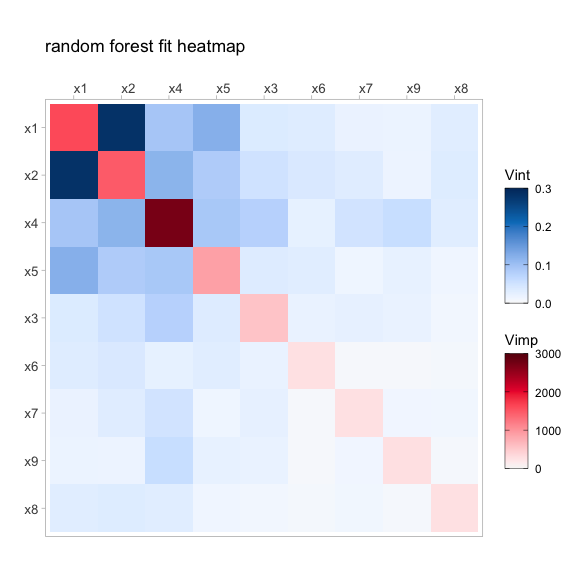Fig 1.0: Heatmap of a random forest fit displaying 2-way interaction strength on the off diagonal and individual variable importance on the diagonal. $$x_1$$ and $$x_2$$ show a strong interaction with $$x_4$$ being the most important for predicting $$y$$.

### Network plot

To create a network graph of the vivi-matrix, we use:

viviNetwork(mat = viFit)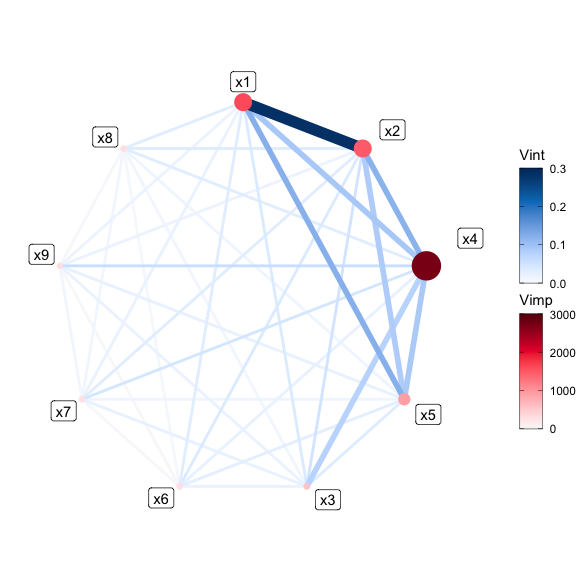Fig 1.1: Network plot of a random forest fit displaying 2-way interaction strength and individual variable importance. $$x_1$$ and $$x_2$$ show a strong interaction with $$x_4$$ being the most important for predicting $$y$$.

### Generalized partial dependence pairs plot

In this plot we use a generalized pairs plot matrix style layout (which we call GPDP) to display partial dependence plots (PDPs) in the upper triangle, individual conditional exception curves (along with the aggregated 1-way partial dependence) on the diagonal and a scatterplot in the lower triangle.

To create the plot we supply the model fit to the plotting function.

set.seed(1701)
pdpPairs(data = myData, fit = fit, response = "y", nmax = 50, gridSize = 10)
#> Generating ice/pdp fits... waiting...
#> Finished ice/pdp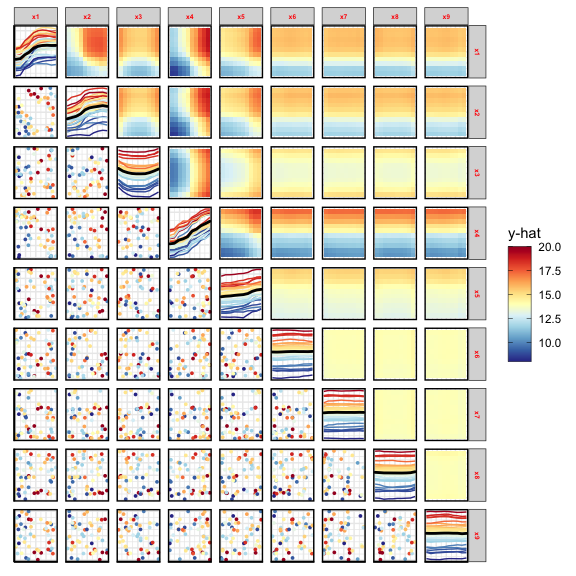Fig 1.3: GPDP of a random forest fit on the Friedman data. From the plot we can see a clear interaction between $$x_1$$ and $$x_2$$. This can be seen in both the changing ICE curves and 2-way PDPs

### Partial dependence ‘Zenplot’

For this plot, we calculate the bivariate partial dependence and display them in a zenplots layout, which we call (ZPDP). The ZPDP is based on graph Eulerians and focuses on key subsets. ‘Zenplots’ create a zigzag expanded navigation plot (‘zenplot’) of the partial dependence values. This results in an alternating sequence of two-dimensional plots laid out in a zigzag structure, as shown in Fig 4.0 below and can be used as a useful space-saving plot that displays the most influential variables.

set.seed(1701)
pdpZen(data = myData, fit = fit, response = "y", nmax = 50, gridSize = 10)
#> Generating ice/pdp fits... waiting...
#> Finished ice/pdp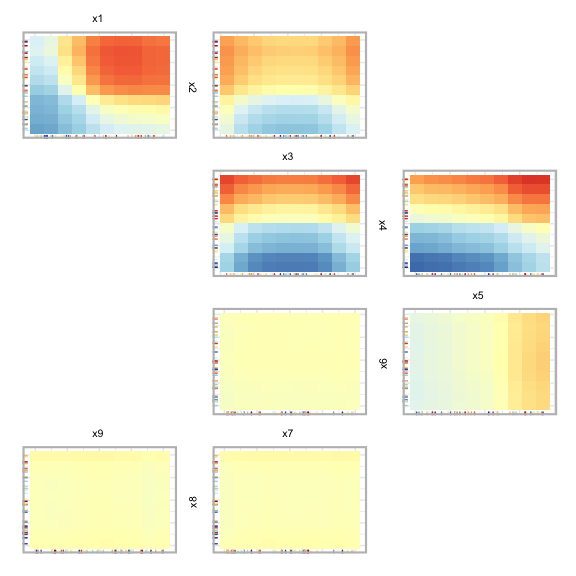Fig 4.0: ZPDP of a random forest fit on a subset of the Friedman data.

In Fig 4.0, we can see PDPs laid out in a zigzag structure, with the most influential variable pairs displayed at the top. As we move down the plot, we also move down in influence of the variable pairs.

Using the zpath argument, we can filter out any interactions below a set value. zpath takes the vivi matrix as a function argument and then, using cutoff, we can filter out any interactions below the chosen value. For example:

set.seed(1701)
zpath <- zPath(viv = viFit, cutoff = 0.1)
pdpZen(data = myData, fit = fit, response = "y", nmax = 50, gridSize = 10, zpath = zpath)
#> Generating ice/pdp fits... waiting...
#> Finished ice/pdp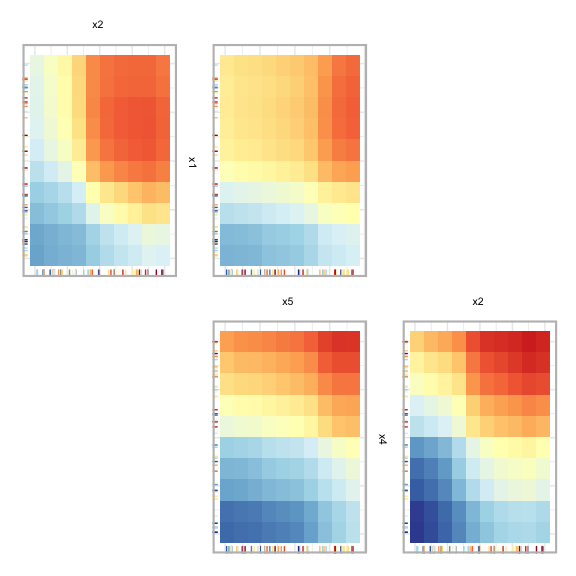Fig 4.1: ZPDP of a random forest fit on a subset of the Friedman data with a zpath of 0.1.

# Section 3: Classification example

In this section, we briefly describe how to apply the above visualisations to a classification example using the iris data set.

To begin we fit a ranger random forest model with “Species” as the response and create the vivi matrix setting the category for classification to be “setosa” using class.

set.seed(1701)
rfClassif <- ranger(Species ~ .,
data = iris, probability = T,
importance = "impurity"
)

set.seed(101)
viviClassif <- vivi(
fit = rfClassif,
data = iris,
response = "Species",
gridSize = 10,
importanceType = NULL,
nmax = 50,
reorder = TRUE,
class = "setosa",
predictFun = NULL
)
#> Embedded impurity variable importance method used.
#> Calculating interactions...

Next we plot the heatmap and network plot of the iris data.

set.seed(1701)
viviHeatmap(mat = viviClassif)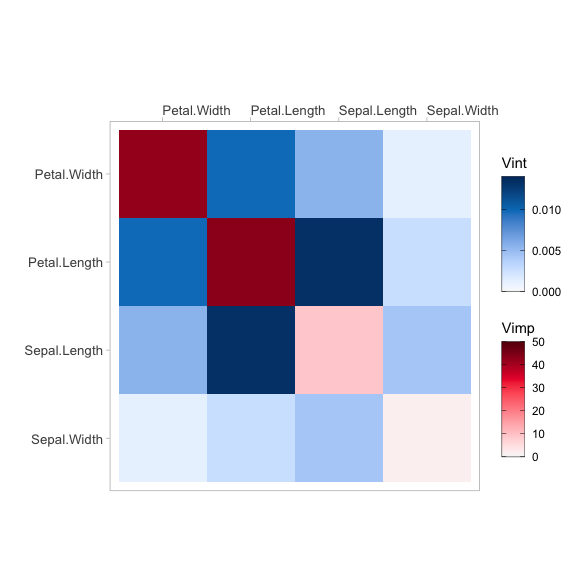Fig 5.0: Heatmap of random forest fit on the iris data.
set.seed(1701)
viviNetwork(mat = viviClassif)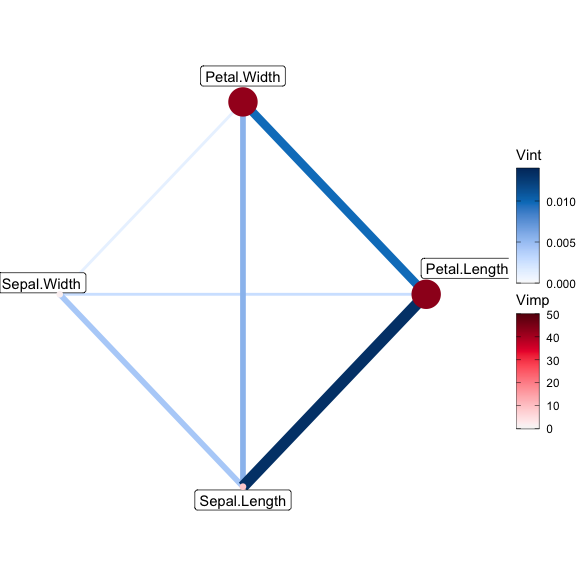Fig 5.1: Network graph of random forest fit on the iris data.

As PDPs are evaluated on a grid, they can extrapolate where there is no data. To solve this issue we calculate a convex hull around the data and remove any points that fall outside the convex hull. This can be seen in the GPDP in Fig 3.2 below.

set.seed(1701)
pdpPairs(data = iris, fit = rfClassif, response = "Species", class = "setosa", convexHull = T,  gridSize = 10, nmax = 50)
#> Generating ice/pdp fits... waiting...
#> Finished ice/pdp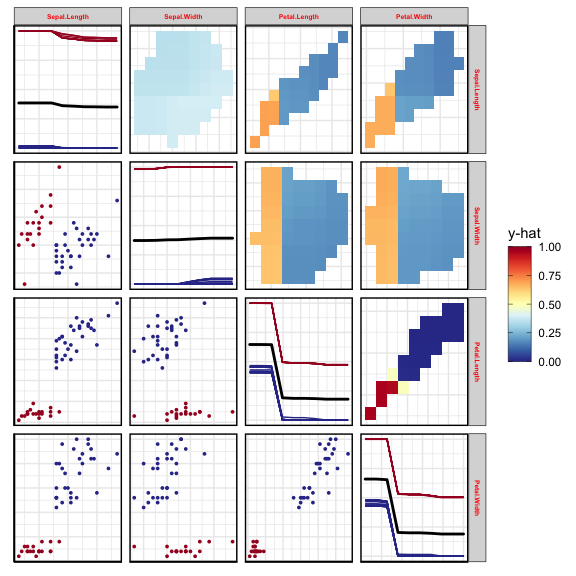Fig 5.2 GPDP of random forest fit on the iris data with extrapolated data removed.

Finally, a ZPDP for the random forest fit on the iris data with extrapolated data removed:

set.seed(1701)
pdpZen(data = iris, fit = rfClassif, response = "Species", class = "setosa", convexHull = T,  gridSize = 10, nmax = 50)
#> Generating ice/pdp fits... waiting...
#> Finished ice/pdp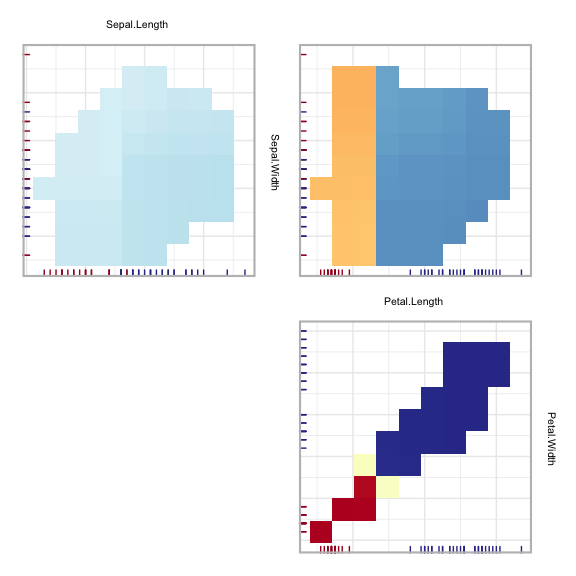Fig 5.2 ZPDP of random forest fit on the iris data with extrapolated data removed.

1. Friedman, Jerome H. (1991) Multivariate adaptive regression splines. The Annals of Statistics 19 (1), pages 1-67.